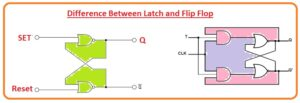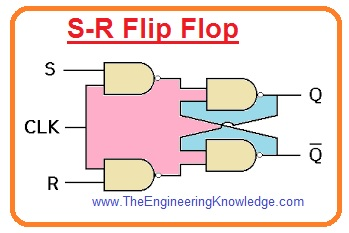Hello fellows, I hope you all are doing great. In today’s tutorial, we will have a look at Difference Between Latch and Flip Flop. The latches and flip-flops are called basic elements of electronic networks. latch varies its output according to a given input signal when it is enabled. While in flip flop the output varies according to conjunction input with the clock signal. In simple words, the clock signal is working as a control signal to show the output according to variation in the input.

As we know that memory chips, logic circuits, and microprocessors are digital ICs that store and send information in the shape of bits. So these called basic building blocks of electronic network. Due to this latch and flip flop are known as derived series circuitry which works for storage of information. In today’s post, we will have a detailed look at both latch and flip flops and compare them to find their differences. So let’s get started with Difference Between Latch and Flip Flop.

#### Difference Between Latch and Flip Flop

Latch

• Its working principle depends on the level triggering.
• It is constructed with the logic gates.
• Its working depends on the applied inputs, applying input, and given output value in binary numbers.
• When it is enabled show sensitivity for an applied signal.
• There is less power required to operate it.
• Its operating speed is high.
• Most common types of Latches are S-R, J-K, T and D.
• It can not be used as register.
• It need less place for operation.
• there is no need of a clock signal for its operation.
• Its circuit analysis is complicated.
• Its robustness is low.

Flip Flop

• Its working operation depends on the edge triggering technique.
• It constructed with clocks.
• It can not be used as registers.
• It circuit analysis is easy.
• Its operation depends on the applying input, applying inputs, and obtained analog output with the pulses of clock.
• It need a large operating area.
• It required a clock signal.
• It required large power for operation than a latch.
• Its operation speed is low.
• It performed synchronous operations.
• The types of flip flops are S-R, J-K, T and D.#### What is Latch

• The latches are series logic circuitry which is constructed with the use of logic gates and used for storage of information in the form of binary numbers.
• This module is level triggered so function only when it enabled.
• It also called bistable multi vibrator since it has 2 stable states.
• Its operation is that when enable pin out is active then variation in the input, output varied.
• In simple word with the variation in input stored data also varied.
• In below figure you can see the S-R latch logic circuit.• In this configuration when enable signal is on the variation in input changes the stored binary information. Due to this it known as level sensitive.

#### What is Flip Flop

• Flip flops are known as series logic circuitry since their current output depend on the applied input, applying input and obtained output.
• But contrary to latch most important role of in operation of flip-flop is clock.
• Therefore, for flip-flop when input varied from one level to other than the stored bits varied only when the clock is changed from one level to other.
• In below figure you can see the S-R flip flop.
• So in simple words flip-flop varies the output with respect to input but with the change in clock signal.
• Therefor clock signal is control signal in this case and therefore the output of flip flop take some time.